« Vrati se

#### IMO Shortlist 2009 problem G4

Given a cyclic quadrilateral$ABCD$, let the diagonals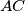$AC$ and$BD$ meet at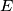$E$ and the lines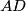$AD$ and$BC$ meet at$F$. The midpoints of$AB$ and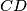$CD$ are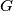$G$ and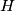$H$, respectively. Show that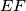$EF$ is tangent at$E$ to the circle through the points$E$,$G$ and$H$.

Proposed by David Monk, United Kingdom